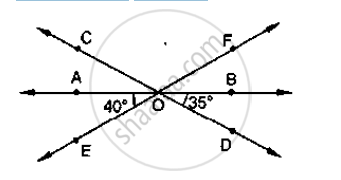# In the Below Fig, Lines Ab, Cd and Ef Intersect at O. Find the Measures of ∠Aoc, ∠Cof, ∠Doe and ∠Bof. - Mathematics

In the below fig, lines AB, CD and EF intersect at O. Find the measures of ∠AOC, ∠COF,
∠DOE and ∠BOF.#### Solution

∠AOE and ∠EOB are linear pair of angles

∠AOE + ∠EOB = 180°

∠AOE + ∠DOE + ∠BOD = 180°

⇒ ∠DOE = 180° - 40° - 35° = 105°

Vertically opposite side angles are equal

∠DOE = ∠COF = 105°

Now, ∠AOE + ∠AOF = 180°                [∵ Linear pair]

⇒  ∠AOE + ∠AOC + ∠COF = 180°

⇒ 40° + ∠AOC +105° = 180°

⇒ ∠AOC = 180° -145°

∠AOC = 35°

Also, ∠BOF = ∠AOE = 40°                    [  ∵ Vertically opposite angle are equal]

Concept: Concept of Parallel Lines
Is there an error in this question or solution?

#### APPEARS IN

RD Sharma Mathematics for Class 9
Chapter 10 Lines and Angles
Exercise 10.3 | Q 7 | Page 23